# RD Sharma Solutions for Class 11 Maths Chapter 14 Quadratic Equations

## RD Sharma Solutions Class 11 Maths Chapter 14 – Download Free PDF Updated Session 2021 – 22

RD Sharma Solutions for Class 11 Maths Chapter 14 – Quadratic Equations are provided here to help students study and score good marks in the board exams. In earlier classes, we have studied quadratic equations with real coefficients and real roots only. In this chapter, we shall study quadratic equations with real coefficients and complex roots. We shall also discuss quadratic equations with complex coefficients and their solutions in the complex number system.

For a better understanding of the concepts, students can solve the exercise wise problems using the RD Sharma Solutions, which are developed by our expert faculty team at BYJU’S. Students aspiring to secure high marks in their examination are advised to practice the solutions on a regular basis. RD Sharma Class 11 Maths Solutions pdf can be downloaded from the links provided below.

Chapter 14 – Quadratic Equations contains two exercises and the RD Sharma Solutions present in this page provide solutions to the questions present in each exercise. Now, let us have a look at the concepts discussed in this chapter.

• Some useful definitions and results.
• Quadratic equations with real coefficients.
• Quadratic equations with complex coefficients.

## Download the pdf of RD Sharma Solutions for Class 11 Maths Chapter 14 – Quadratic Equations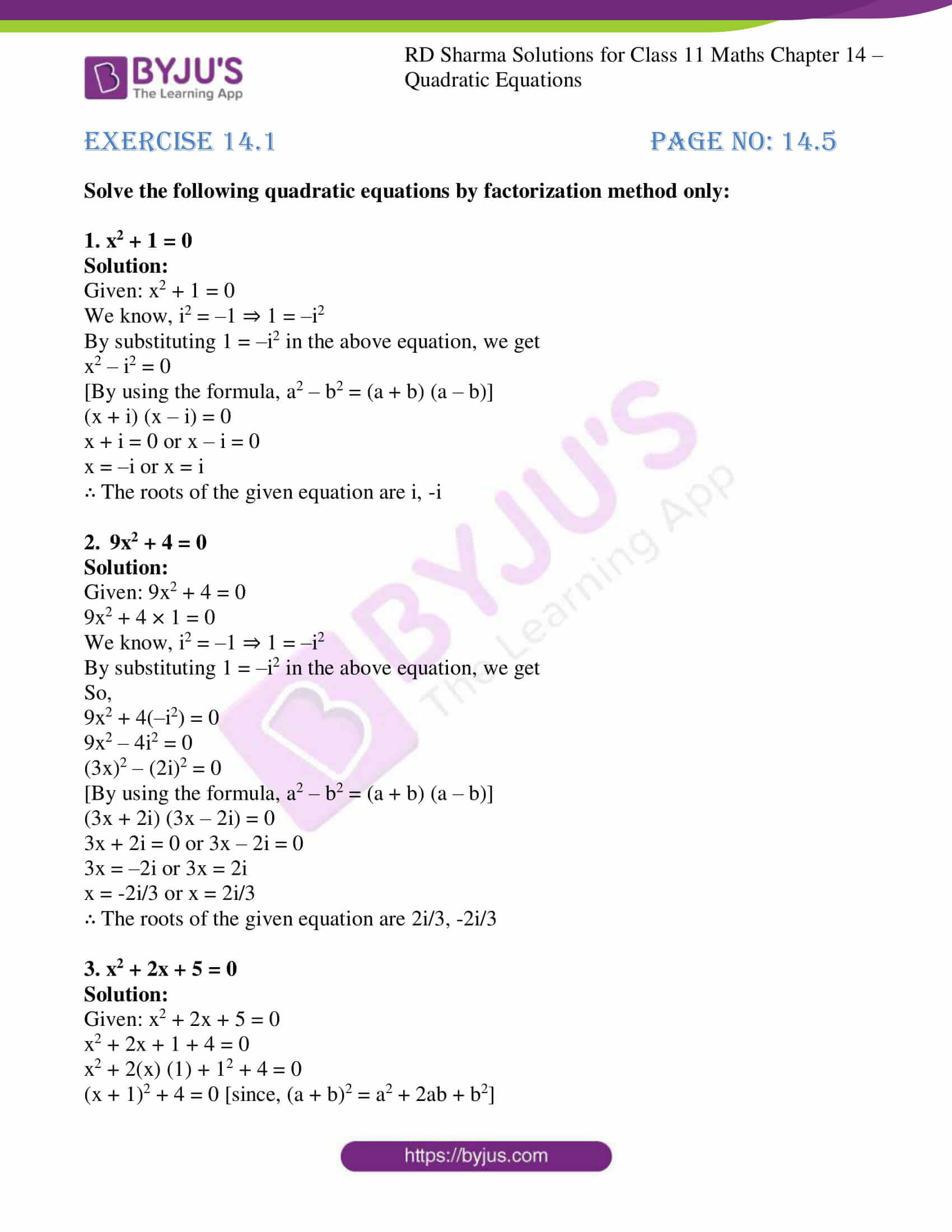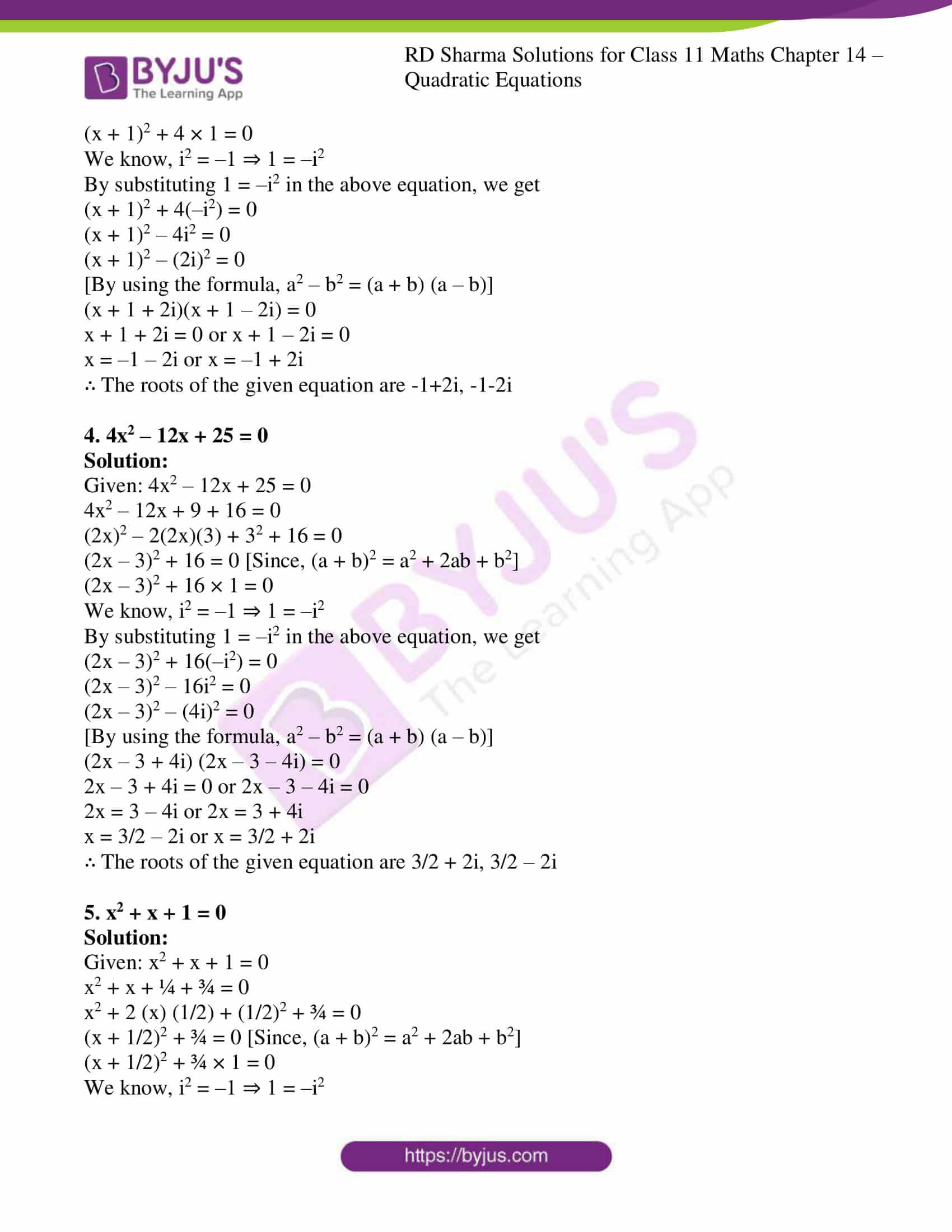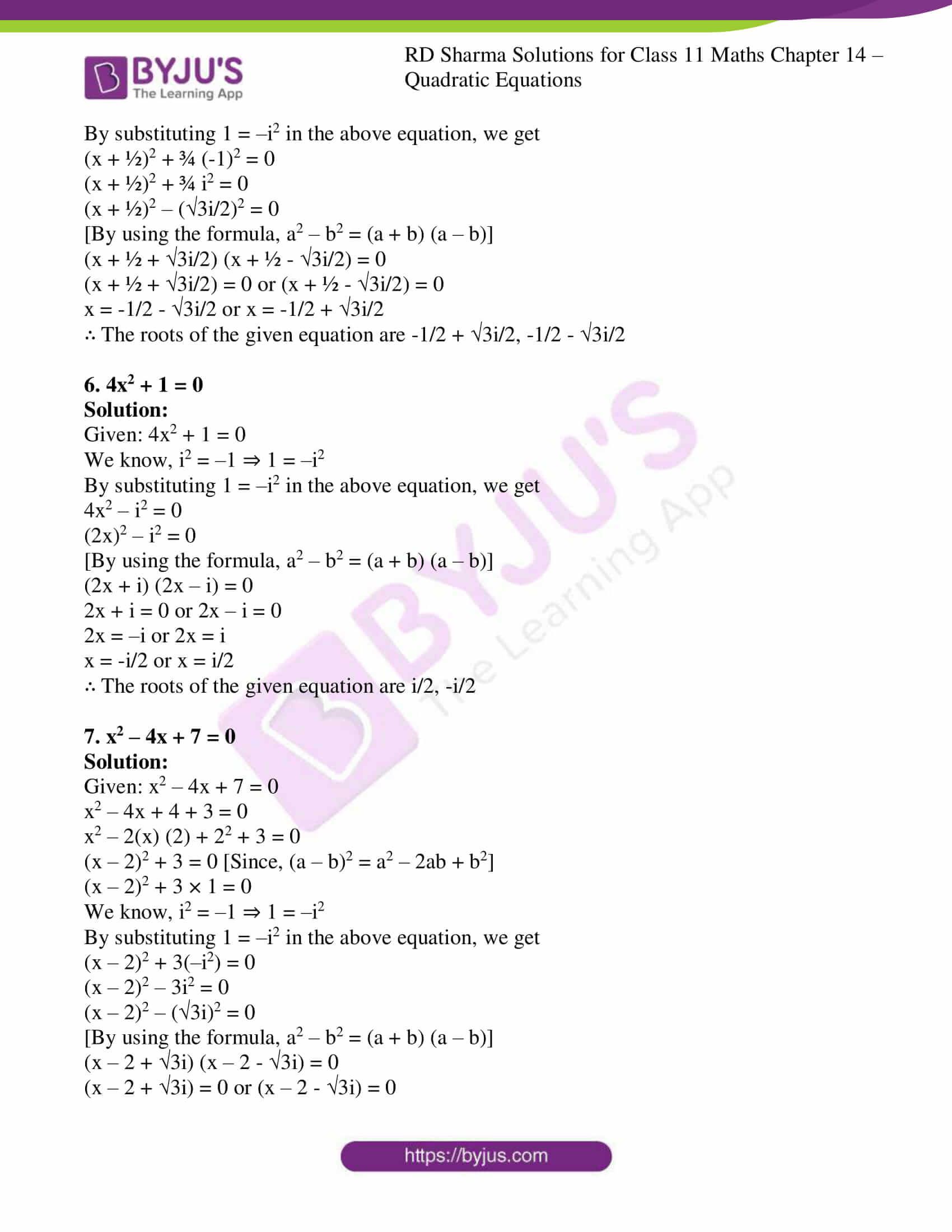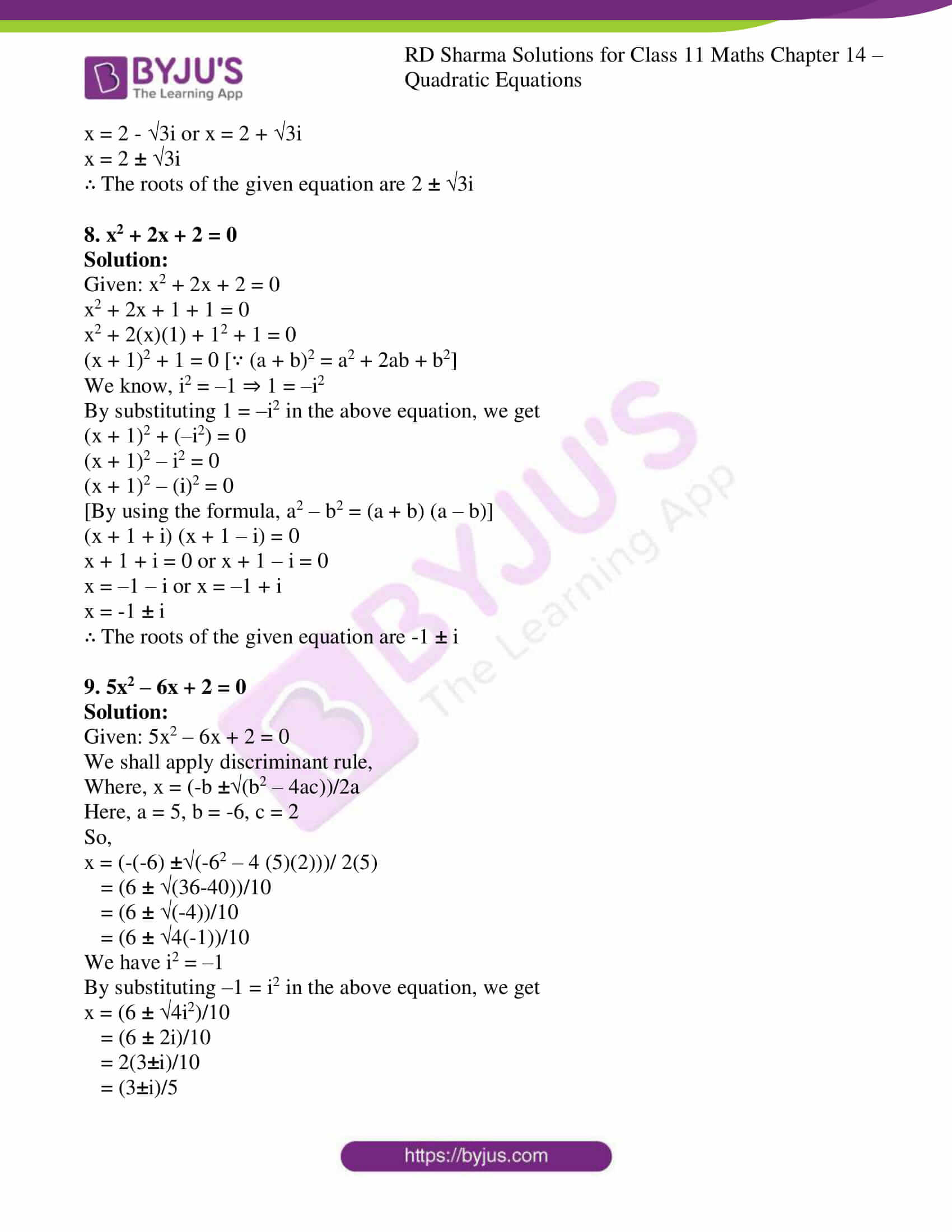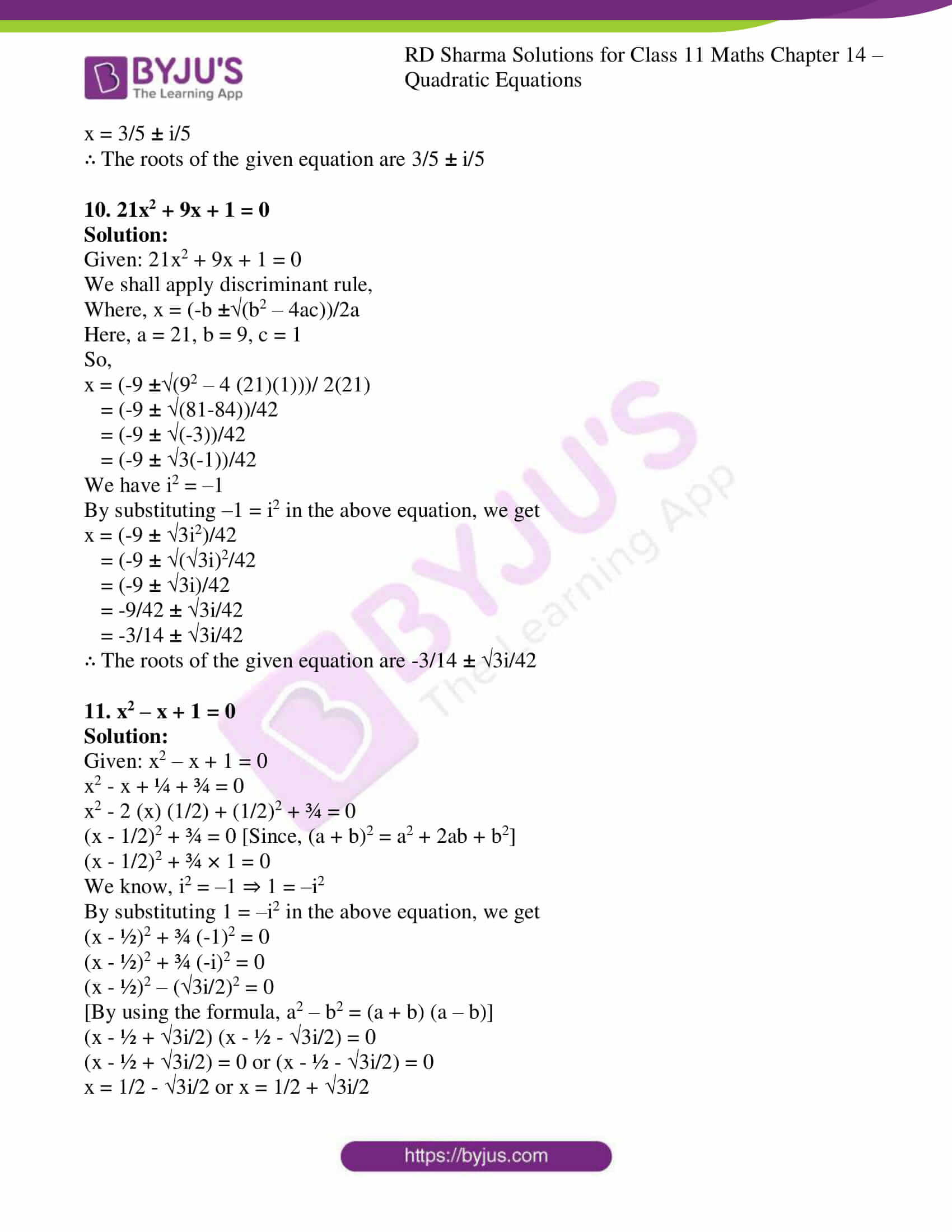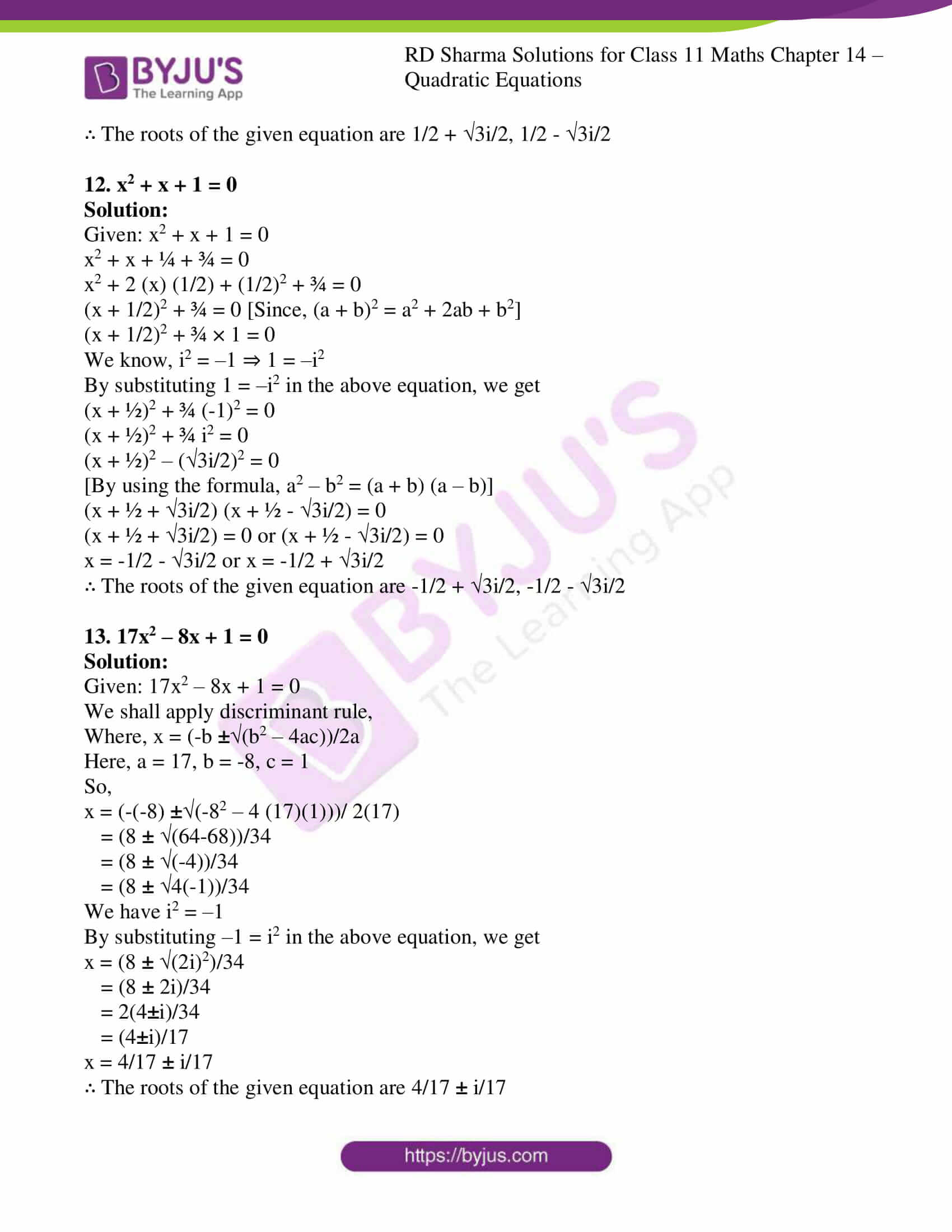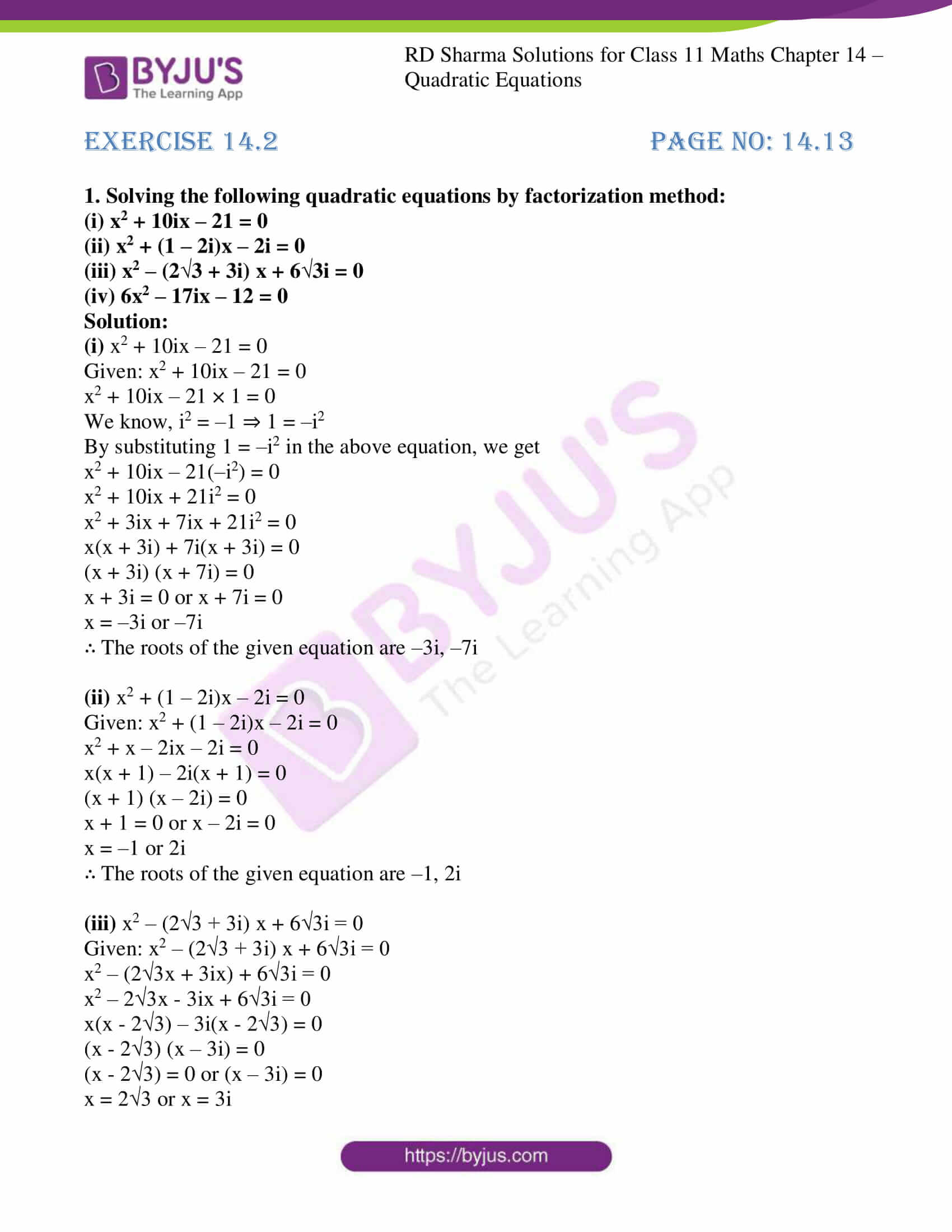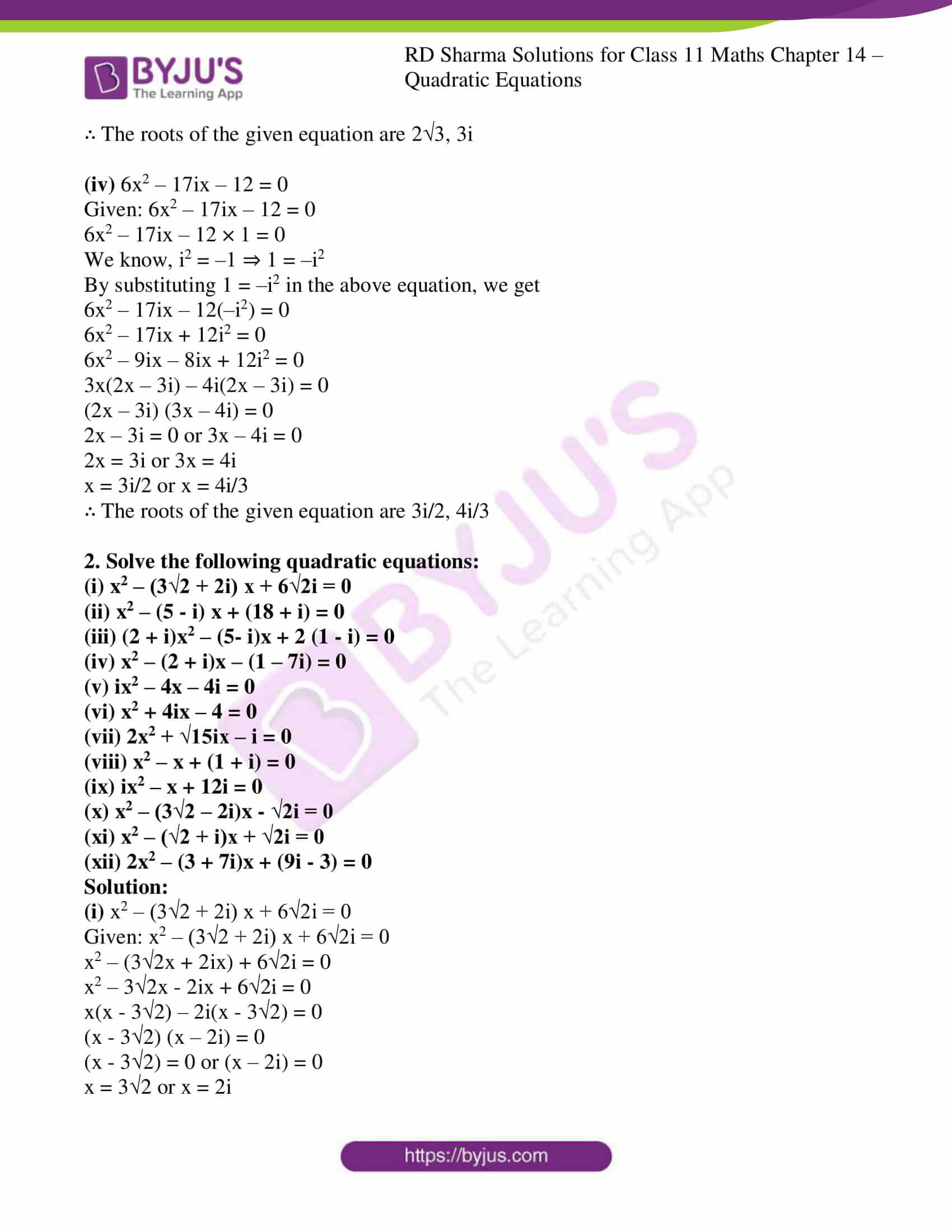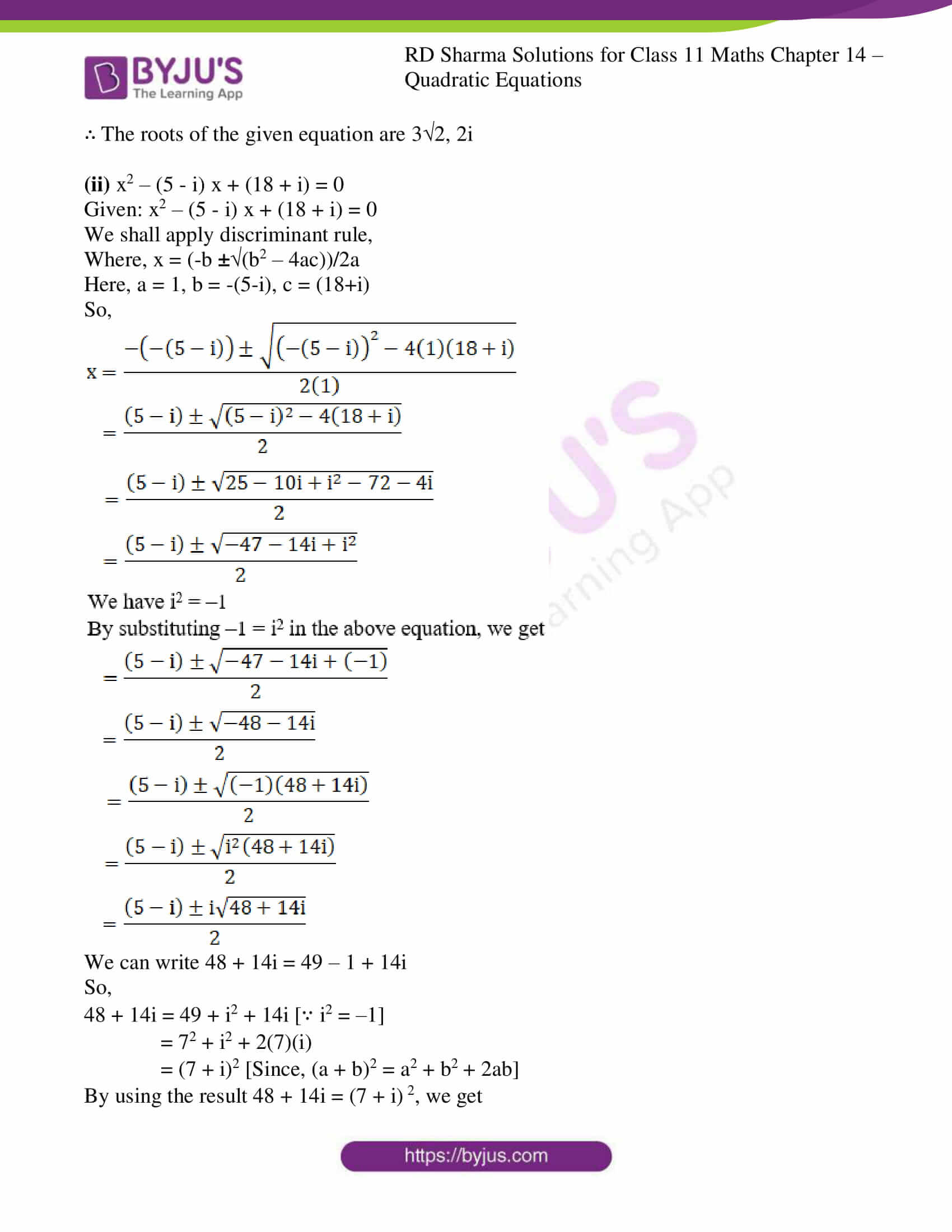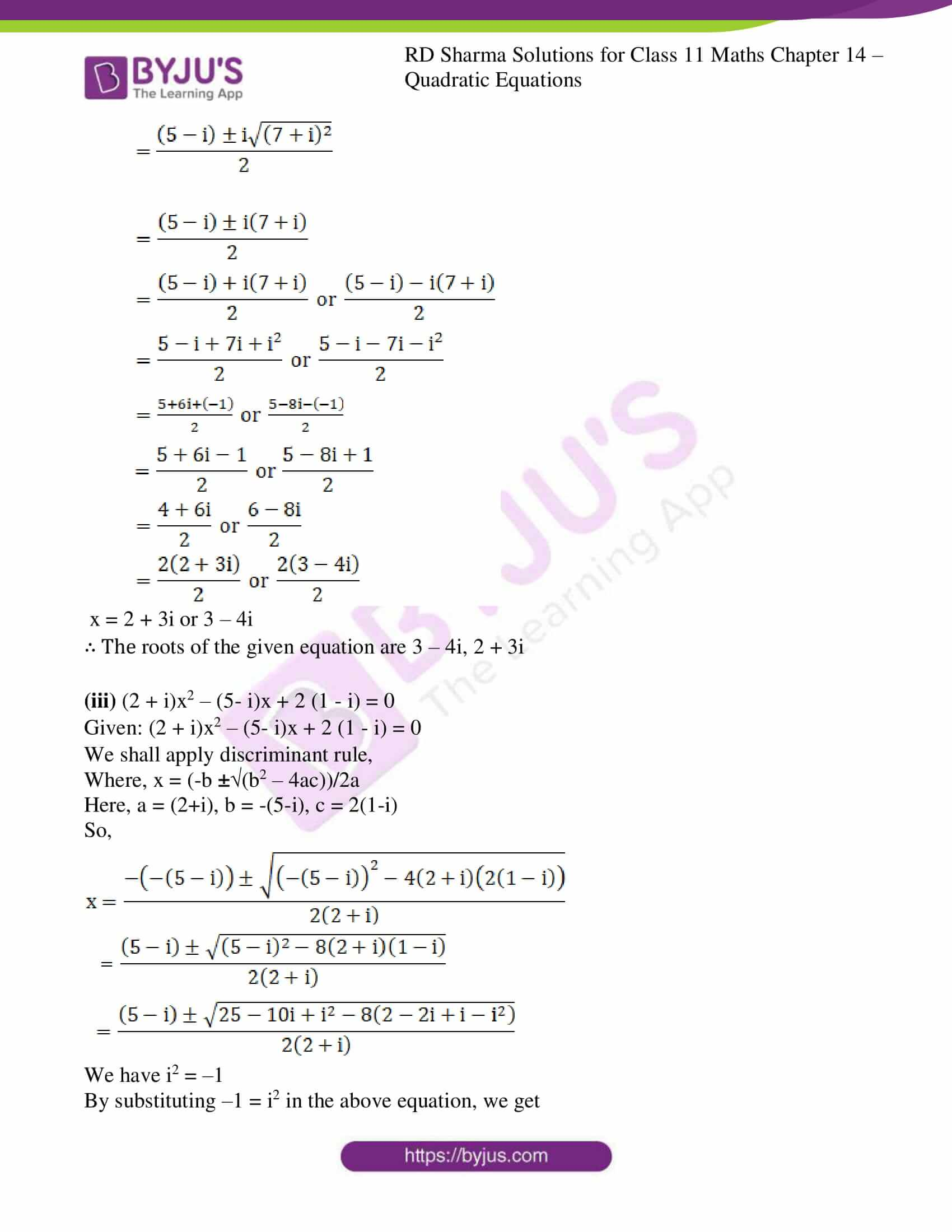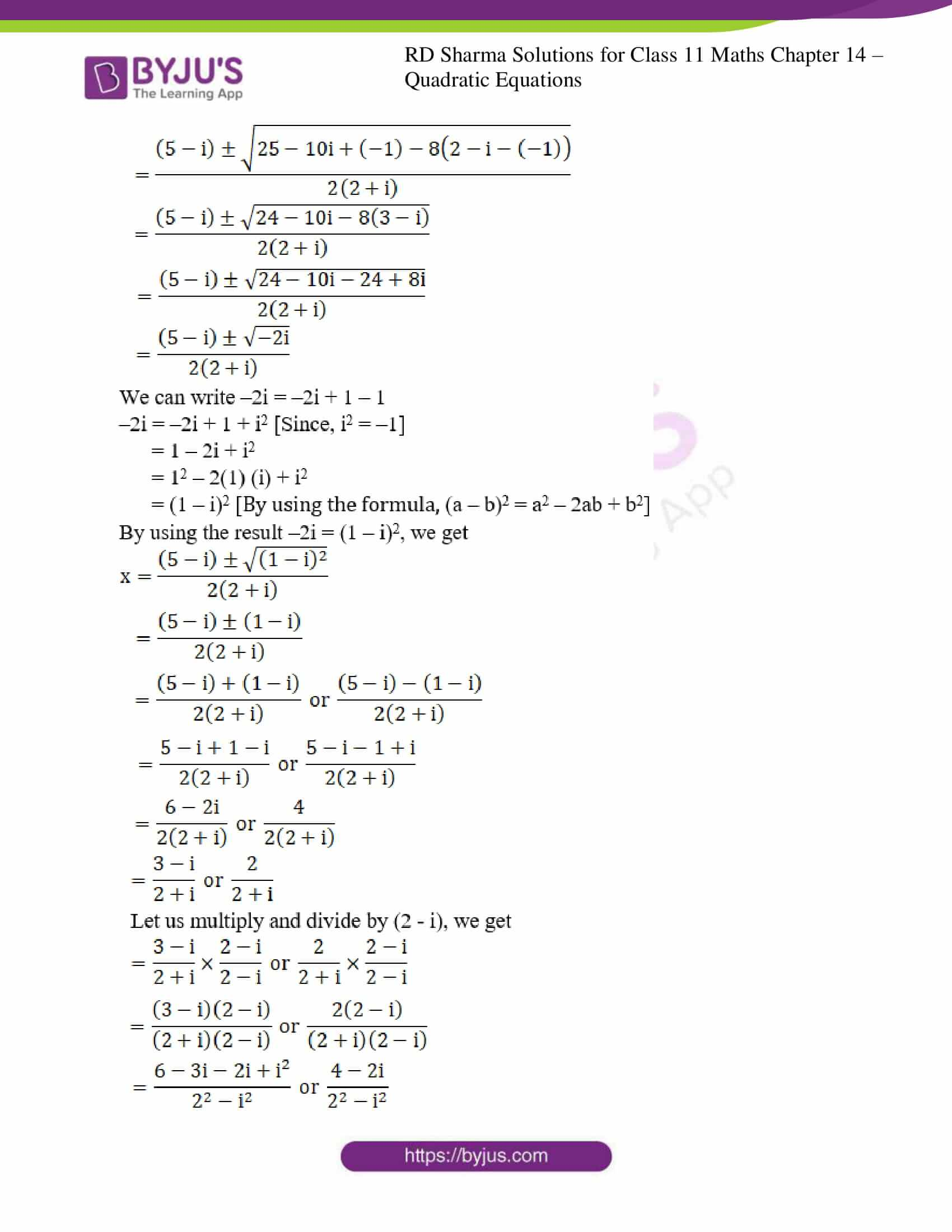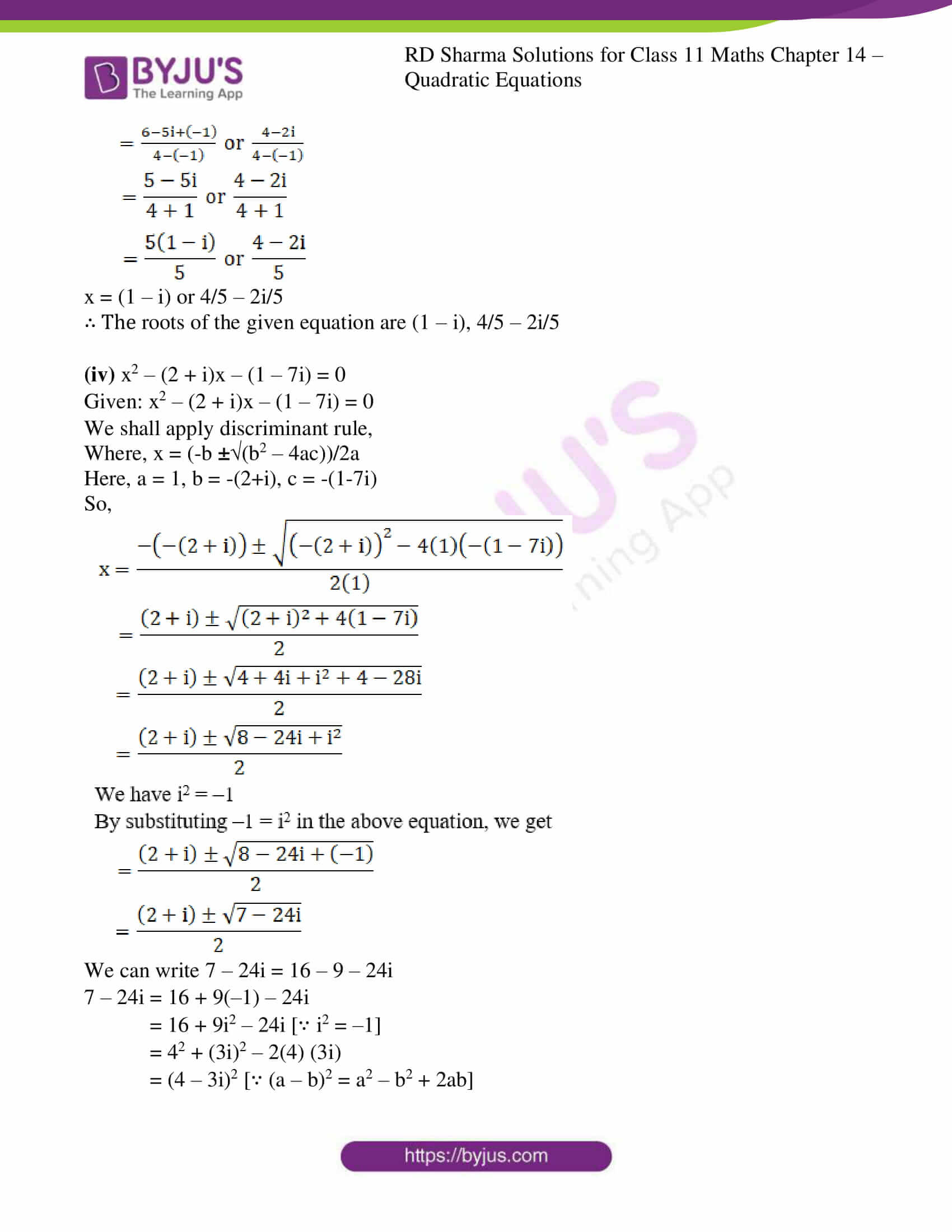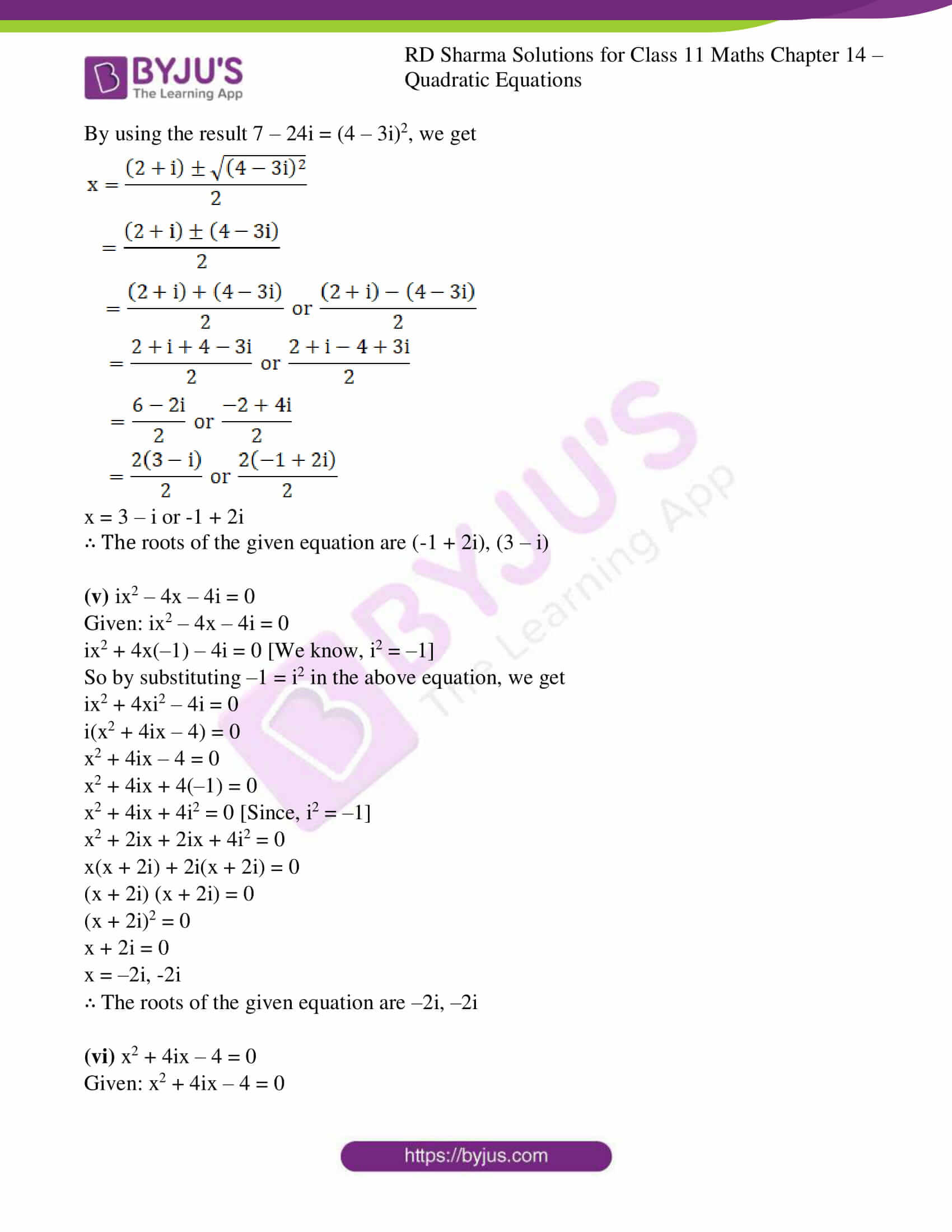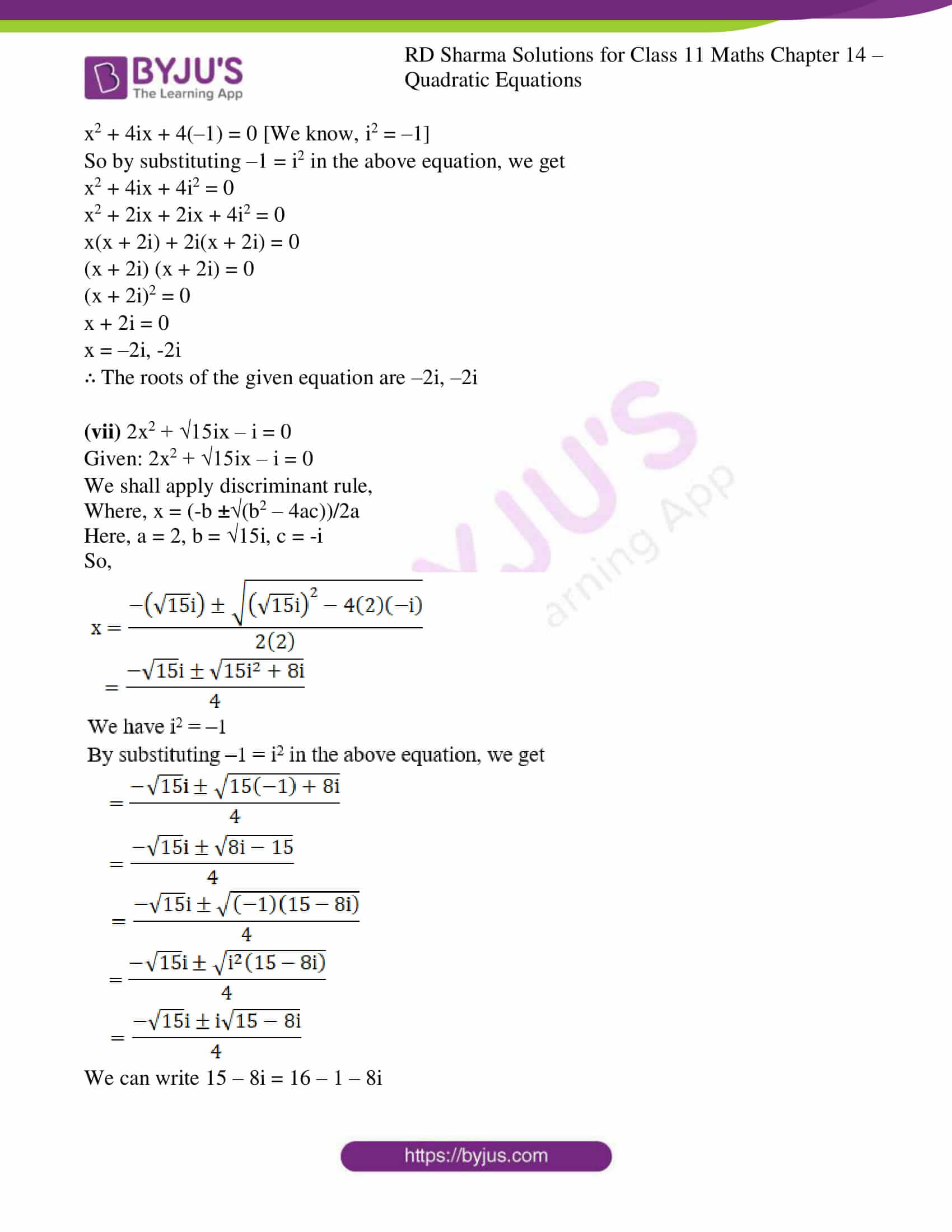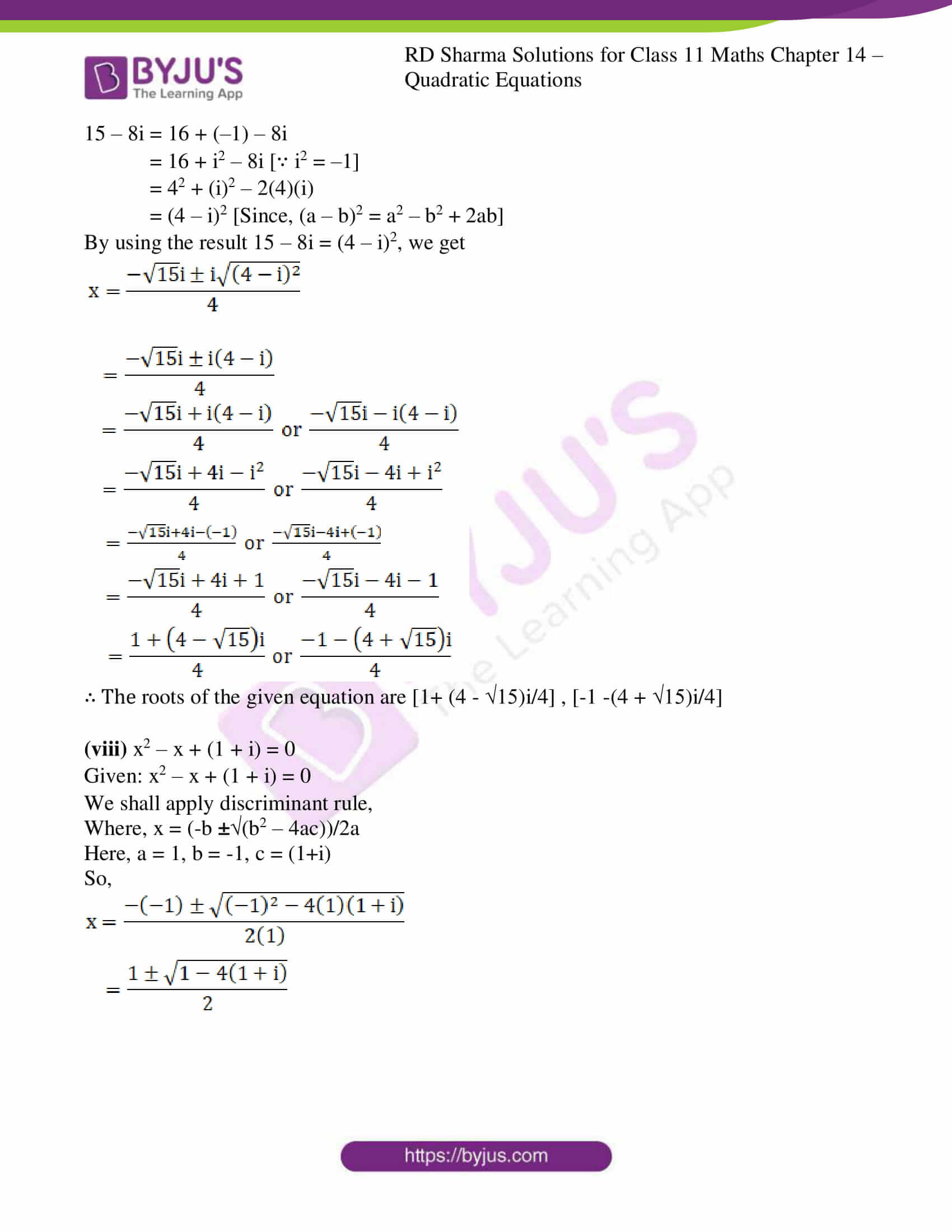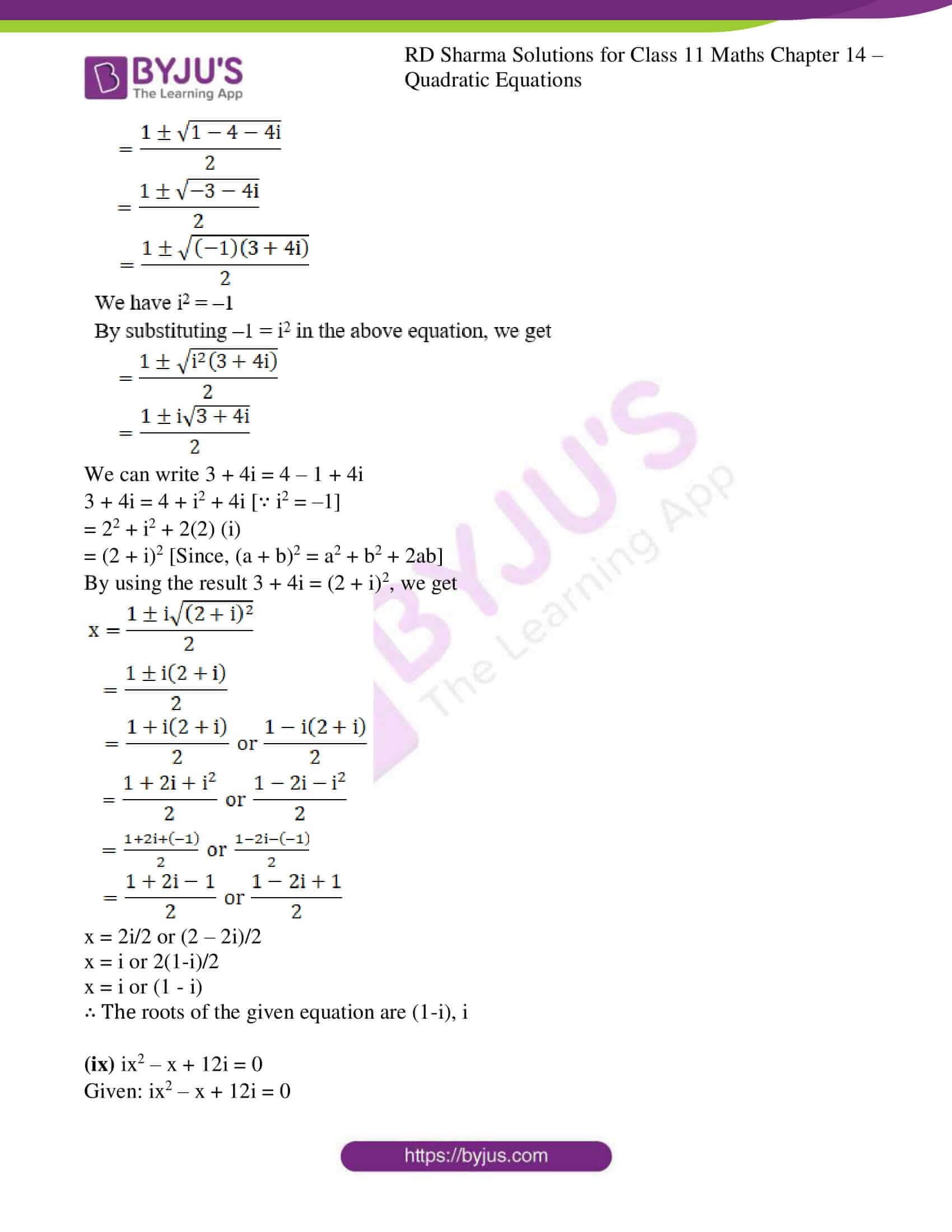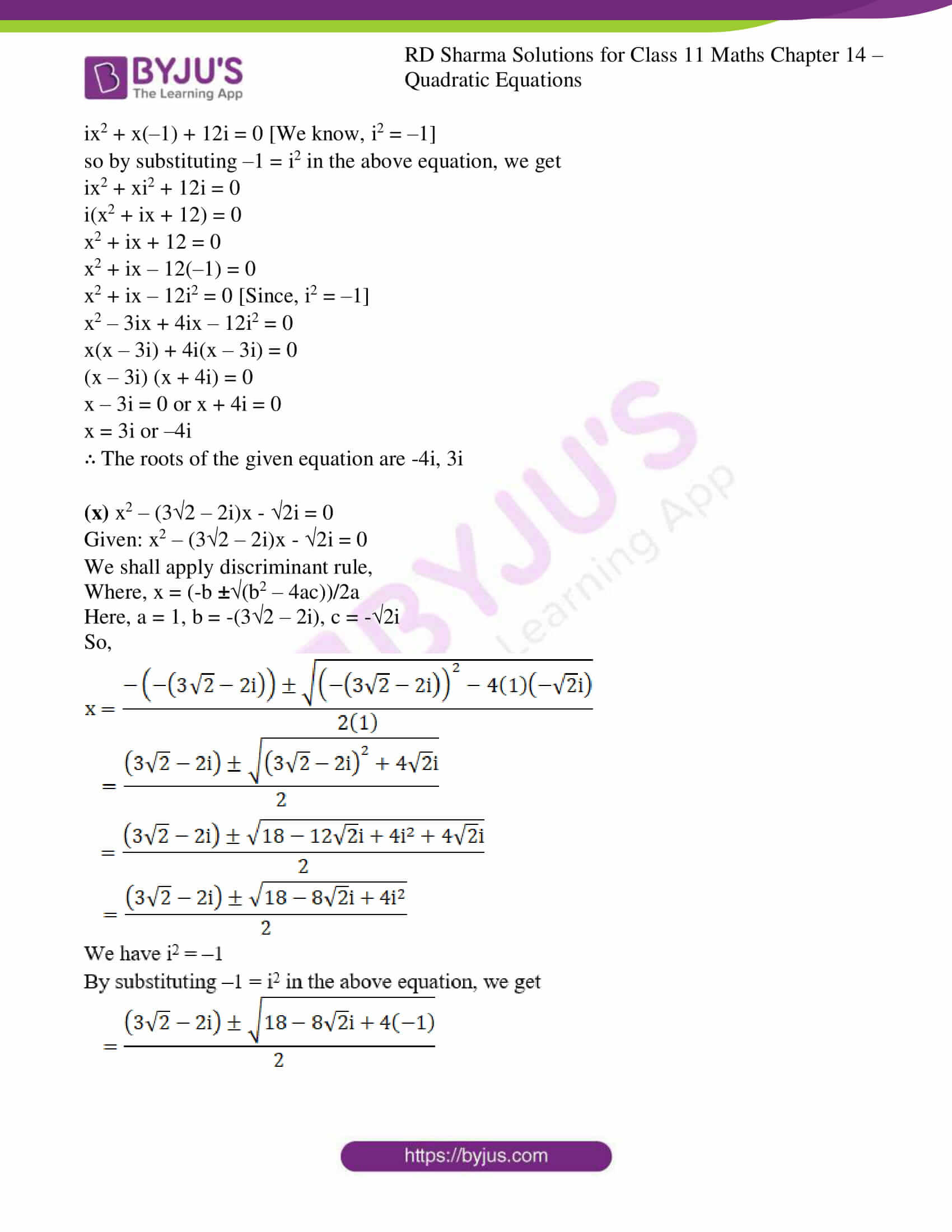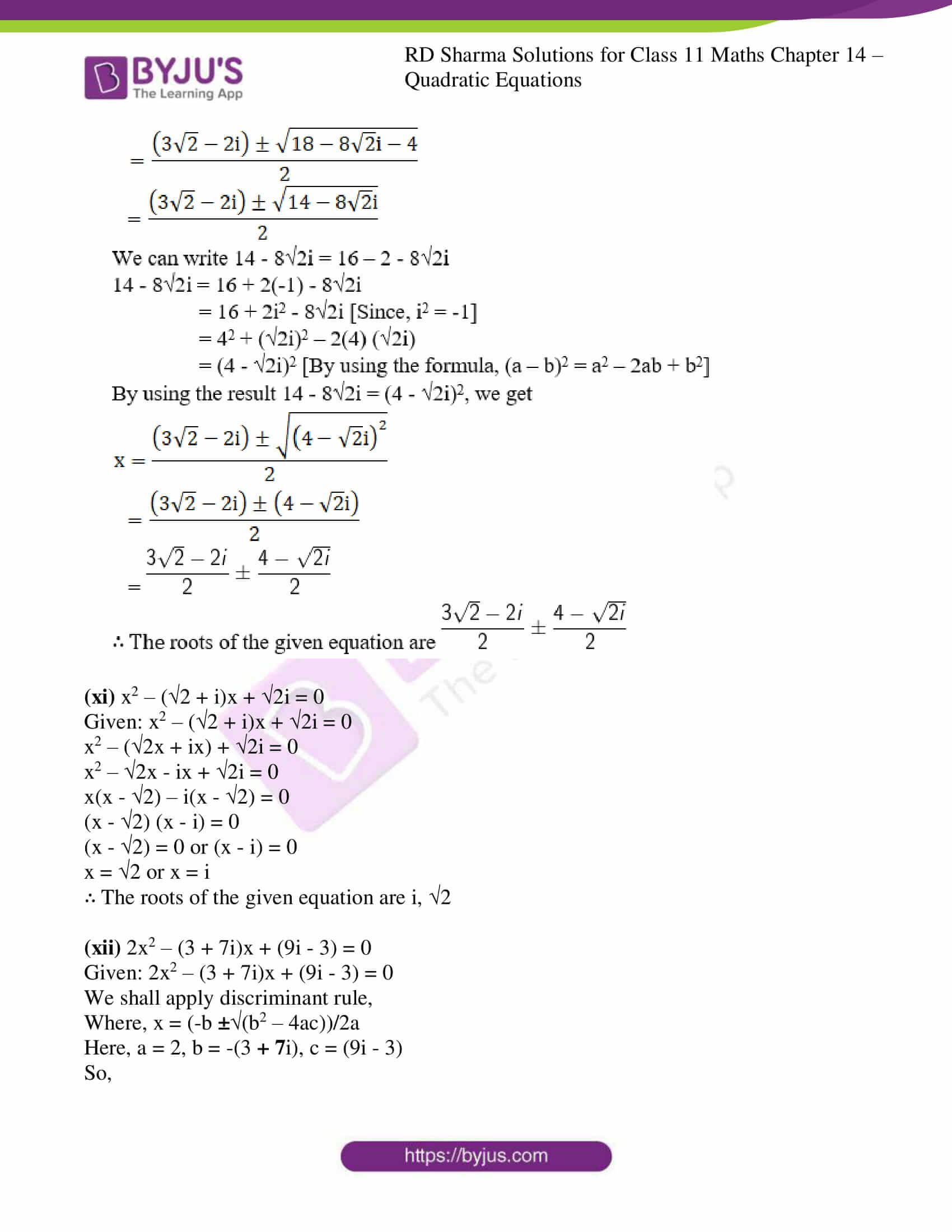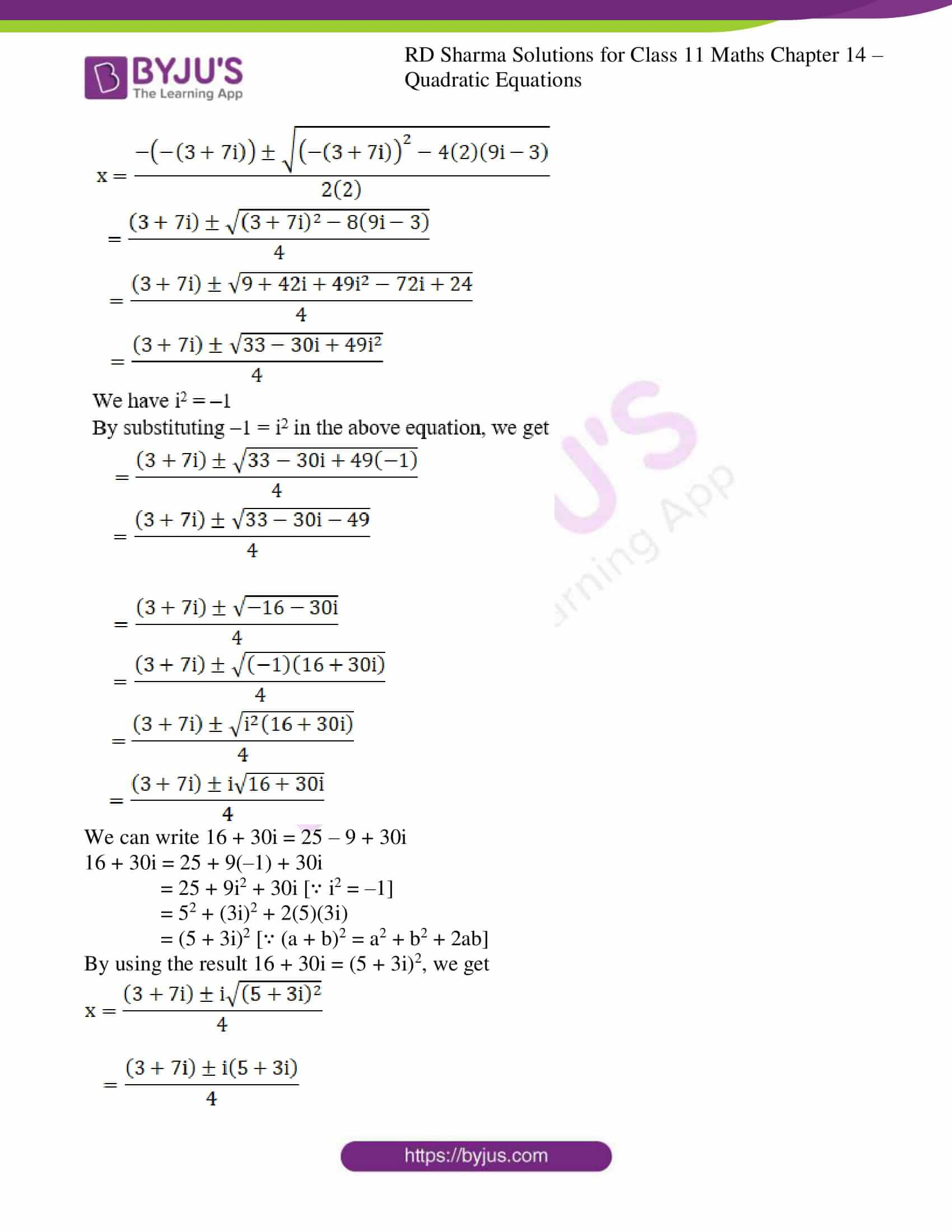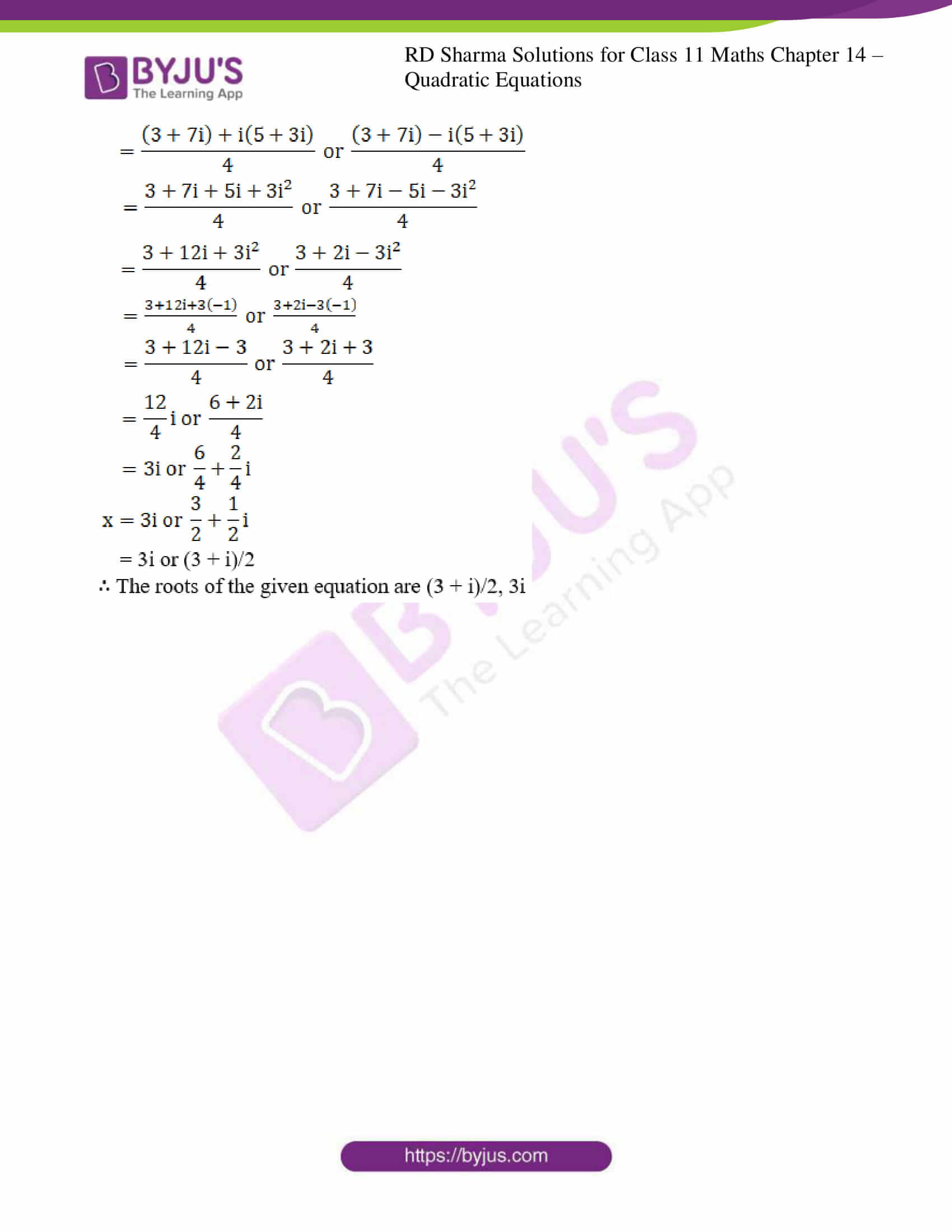### Access answers to RD Sharma Solutions for Class 11 Maths Chapter 14 – Quadratic Equations

EXERCISE 14.1 PAGE NO: 14.5

Solve the following quadratic equations by factorization method only:

1. x2 + 1 = 0

Solution:

Given: x2 + 1 = 0

We know, i2 = –1 ⇒ 1 = –i2

By substituting 1 = –i2 in the above equation, we get

x2 – i2 = 0

[By using the formula, a2 – b2 = (a + b) (a – b)]

(x + i) (x – i) = 0

x + i = 0 or x – i = 0

x = –i or x = i

∴ The roots of the given equation are i, -i

2. 9x2 + 4 = 0

Solution:

Given: 9x2 + 4 = 0

9x2 + 4 × 1 = 0

We know, i2 = –1 ⇒ 1 = –i2

By substituting 1 = –i2 in the above equation, we get

So,

9x2 + 4(–i2) = 0

9x2 – 4i2 = 0

(3x)2 – (2i)2 = 0

[By using the formula, a2 – b2 = (a + b) (a – b)]

(3x + 2i) (3x – 2i) = 0

3x + 2i = 0 or 3x – 2i = 0

3x = –2i or 3x = 2i

x = -2i/3 or x = 2i/3

∴ The roots of the given equation are 2i/3, -2i/3

3. x2 + 2x + 5 = 0

Solution:

Given: x2 + 2x + 5 = 0

x2 + 2x + 1 + 4 = 0

x2 + 2(x) (1) + 12 + 4 = 0

(x + 1)2 + 4 = 0 [since, (a + b)2 = a2 + 2ab + b2]

(x + 1)2 + 4 × 1 = 0

We know, i2 = –1 ⇒ 1 = –i2

By substituting 1 = –i2 in the above equation, we get

(x + 1)2 + 4(–i2) = 0

(x + 1)2 – 4i2 = 0

(x + 1)2 – (2i)2 = 0

[By using the formula, a2 – b2 = (a + b) (a – b)]

(x + 1 + 2i)(x + 1 – 2i) = 0

x + 1 + 2i = 0 or x + 1 – 2i = 0

x = –1 – 2i or x = –1 + 2i

∴ The roots of the given equation are -1+2i, -1-2i

4. 4x2 – 12x + 25 = 0

Solution:

Given: 4x2 – 12x + 25 = 0

4x2 – 12x + 9 + 16 = 0

(2x)2 – 2(2x)(3) + 32 + 16 = 0

(2x – 3)2 + 16 = 0 [Since, (a + b)2 = a2 + 2ab + b2]

(2x – 3)2 + 16 × 1 = 0

We know, i2 = –1 ⇒ 1 = –i2

By substituting 1 = –i2 in the above equation, we get

(2x – 3)2 + 16(–i2) = 0

(2x – 3)2 – 16i2 = 0

(2x – 3)2 – (4i)2 = 0

[By using the formula, a2 – b2 = (a + b) (a – b)]

(2x – 3 + 4i) (2x – 3 – 4i) = 0

2x – 3 + 4i = 0 or 2x – 3 – 4i = 0

2x = 3 – 4i or 2x = 3 + 4i

x = 3/2 – 2i or x = 3/2 + 2i

∴ The roots of the given equation are 3/2 + 2i, 3/2 – 2i

5. x2 + x + 1 = 0

Solution:

Given: x2 + x + 1 = 0

x2 + x + ¼ + ¾ = 0

x2 + 2 (x) (1/2) + (1/2)2 + ¾ = 0

(x + 1/2)2 + ¾ = 0 [Since, (a + b)2 = a2 + 2ab + b2]

(x + 1/2)2 + ¾ × 1 = 0

We know, i2 = –1 ⇒ 1 = –i2

By substituting 1 = –i2 in the above equation, we get

(x + ½)2 + ¾ (-1)2 = 0

(x + ½)2 + ¾ i2 = 0

(x + ½)2 – (3i/2)2 = 0

[By using the formula, a2 – b2 = (a + b) (a – b)]

(x + ½ + 3i/2) (x + ½ – 3i/2) = 0

(x + ½ + 3i/2) = 0 or (x + ½ – 3i/2) = 0

x = -1/2 – 3i/2 or x = -1/2 + 3i/2

∴ The roots of the given equation are -1/2 + 3i/2, -1/2 – 3i/2

6. 4x2 + 1 = 0

Solution:

Given: 4x2 + 1 = 0

We know, i2 = –1 ⇒ 1 = –i2

By substituting 1 = –i2 in the above equation, we get

4x2 – i2 = 0

(2x)2 – i2 = 0

[By using the formula, a2 – b2 = (a + b) (a – b)]

(2x + i) (2x – i) = 0

2x + i = 0 or 2x – i = 0

2x = –i or 2x = i

x = -i/2 or x = i/2

∴ The roots of the given equation are i/2, -i/2

7. x2 – 4x + 7 = 0

Solution:

Given: x2 – 4x + 7 = 0

x2 – 4x + 4 + 3 = 0

x2 – 2(x) (2) + 22 + 3 = 0

(x – 2)2 + 3 = 0 [Since, (a – b)2 = a2 – 2ab + b2]

(x – 2)2 + 3 × 1 = 0

We know, i2 = –1 ⇒ 1 = –i2

By substituting 1 = –i2 in the above equation, we get

(x – 2)2 + 3(–i2) = 0

(x – 2)2 – 3i2 = 0

(x – 2)2 – (3i)2 = 0

[By using the formula, a2 – b2 = (a + b) (a – b)]

(x – 2 + 3i) (x – 2 – 3i) = 0

(x – 2 + 3i) = 0 or (x – 2 – 3i) = 0

x = 2 – 3i or x = 2 + 3i

x = 2 ± 3i

∴ The roots of the given equation are 2 ± 3i

8. x2 + 2x + 2 = 0

Solution:

Given: x2 + 2x + 2 = 0

x2 + 2x + 1 + 1 = 0

x2 + 2(x)(1) + 12 + 1 = 0

(x + 1)2 + 1 = 0 [∵ (a + b)2 = a2 + 2ab + b2]

We know, i2 = –1 ⇒ 1 = –i2

By substituting 1 = –i2 in the above equation, we get

(x + 1)2 + (–i2) = 0

(x + 1)2 – i2 = 0

(x + 1)2 – (i)2 = 0

[By using the formula, a2 – b2 = (a + b) (a – b)]

(x + 1 + i) (x + 1 – i) = 0

x + 1 + i = 0 or x + 1 – i = 0

x = –1 – i or x = –1 + i

x = -1 ± i

∴ The roots of the given equation are -1 ± i

9. 5x2 – 6x + 2 = 0

Solution:

Given: 5x2 – 6x + 2 = 0

We shall apply discriminant rule,

Where, x = (-b ±√(b2 – 4ac))/2a

Here, a = 5, b = -6, c = 2

So,

x = (-(-6) ±√(-62 – 4 (5)(2)))/ 2(5)

= (6 ± √(36-40))/10

= (6 ± √(-4))/10

= (6 ± √4(-1))/10

We have i2 = –1

By substituting –1 = i2 in the above equation, we get

x = (6 ± √4i2)/10

= (6 ± 2i)/10

= 2(3±i)/10

= (3±i)/5

x = 3/5 ± i/5

∴ The roots of the given equation are 3/5 ± i/5

10. 21x2 + 9x + 1 = 0

Solution:

Given: 21x2 + 9x + 1 = 0

We shall apply discriminant rule,

Where, x = (-b ±√(b2 – 4ac))/2a

Here, a = 21, b = 9, c = 1

So,

x = (-9 ±√(92 – 4 (21)(1)))/ 2(21)

= (-9 ± √(81-84))/42

= (-9 ± √(-3))/42

= (-9 ± √3(-1))/42

We have i2 = –1

By substituting –1 = i2 in the above equation, we get

x = (-9 ± √3i2)/42

= (-9 ± √(3i)2/42

= (-9 ± √3i)/42

= -9/42 ± √3i/42

= -3/14 ± √3i/42

∴ The roots of the given equation are -3/14 ± √3i/42

11. x2 – x + 1 = 0

Solution:

Given: x2 – x + 1 = 0

x2 – x + ¼ + ¾ = 0

x2 – 2 (x) (1/2) + (1/2)2 + ¾ = 0

(x – 1/2)2 + ¾ = 0 [Since, (a + b)2 = a2 + 2ab + b2]

(x – 1/2)2 + ¾ × 1 = 0

We know, i2 = –1 ⇒ 1 = –i2

By substituting 1 = –i2 in the above equation, we get

(x – ½)2 + ¾ (-1)2 = 0

(x – ½)2 + ¾ (-i)2 = 0

(x – ½)2 – (3i/2)2 = 0

[By using the formula, a2 – b2 = (a + b) (a – b)]

(x – ½ + 3i/2) (x – ½ – 3i/2) = 0

(x – ½ + 3i/2) = 0 or (x – ½ – 3i/2) = 0

x = 1/2 – 3i/2 or x = 1/2 + 3i/2

∴ The roots of the given equation are 1/2 + 3i/2, 1/2 – 3i/2

12. x2 + x + 1 = 0

Solution:

Given: x2 + x + 1 = 0

x2 + x + ¼ + ¾ = 0

x2 + 2 (x) (1/2) + (1/2)2 + ¾ = 0

(x + 1/2)2 + ¾ = 0 [Since, (a + b)2 = a2 + 2ab + b2]

(x + 1/2)2 + ¾ × 1 = 0

We know, i2 = –1 ⇒ 1 = –i2

By substituting 1 = –i2 in the above equation, we get

(x + ½)2 + ¾ (-1)2 = 0

(x + ½)2 + ¾ i2 = 0

(x + ½)2 – (3i/2)2 = 0

[By using the formula, a2 – b2 = (a + b) (a – b)]

(x + ½ + 3i/2) (x + ½ – 3i/2) = 0

(x + ½ + 3i/2) = 0 or (x + ½ – 3i/2) = 0

x = -1/2 – 3i/2 or x = -1/2 + 3i/2

∴ The roots of the given equation are -1/2 + 3i/2, -1/2 – 3i/2

13. 17x2 – 8x + 1 = 0

Solution:

Given: 17x2 – 8x + 1 = 0

We shall apply discriminant rule,

Where, x = (-b ±√(b2 – 4ac))/2a

Here, a = 17, b = -8, c = 1

So,

x = (-(-8) ±√(-82 – 4 (17)(1)))/ 2(17)

= (8 ± √(64-68))/34

= (8 ± √(-4))/34

= (8 ± √4(-1))/34

We have i2 = –1

By substituting –1 = i2 in the above equation, we get

x = (8 ± √(2i)2)/34

= (8 ± 2i)/34

= 2(4±i)/34

= (4±i)/17

x = 4/17 ± i/17

∴ The roots of the given equation are 4/17 ± i/17

EXERCISE 14.2 PAGE NO: 14.13

1. Solving the following quadratic equations by factorization method:

(i) x2 + 10ix – 21 = 0

(ii) x2 + (1 – 2i)x – 2i = 0

(iii) x2 – (2√3 + 3i) x + 6√3i = 0

(iv) 6x2 – 17ix – 12 = 0

Solution:

(i) x2 + 10ix – 21 = 0

Given: x2 + 10ix – 21 = 0

x2 + 10ix – 21 × 1 = 0

We know, i2 = –1 ⇒ 1 = –i2

By substituting 1 = –i2 in the above equation, we get

x2 + 10ix – 21(–i2) = 0

x2 + 10ix + 21i2 = 0

x2 + 3ix + 7ix + 21i2 = 0

x(x + 3i) + 7i(x + 3i) = 0

(x + 3i) (x + 7i) = 0

x + 3i = 0 or x + 7i = 0

x = –3i or –7i

∴ The roots of the given equation are –3i, –7i

(ii) x2 + (1 – 2i)x – 2i = 0

Given: x2 + (1 – 2i)x – 2i = 0

x2 + x – 2ix – 2i = 0

x(x + 1) – 2i(x + 1) = 0

(x + 1) (x – 2i) = 0

x + 1 = 0 or x – 2i = 0

x = –1 or 2i

∴ The roots of the given equation are –1, 2i

(iii) x2 – (2√3 + 3i) x + 6√3i = 0

Given: x2 – (2√3 + 3i) x + 6√3i = 0

x2 – (2√3x + 3ix) + 6√3i = 0

x2 – 2√3x – 3ix + 6√3i = 0

x(x – 2√3) – 3i(x – 2√3) = 0

(x – 2√3) (x – 3i) = 0

(x – 2√3) = 0 or (x – 3i) = 0

x = 2√3 or x = 3i

∴ The roots of the given equation are 2√3, 3i

(iv) 6x2 – 17ix – 12 = 0

Given: 6x2 – 17ix – 12 = 0

6x2 – 17ix – 12 × 1 = 0

We know, i2 = –1 ⇒ 1 = –i2

By substituting 1 = –i2 in the above equation, we get

6x2 – 17ix – 12(–i2) = 0

6x2 – 17ix + 12i2 = 0

6x2 – 9ix – 8ix + 12i2 = 0

3x(2x – 3i) – 4i(2x – 3i) = 0

(2x – 3i) (3x – 4i) = 0

2x – 3i = 0 or 3x – 4i = 0

2x = 3i or 3x = 4i

x = 3i/2 or x = 4i/3

∴ The roots of the given equation are 3i/2, 4i/3

2. Solve the following quadratic equations:

(i) x2 – (3√2 + 2i) x + 6√2i = 0

(ii) x2 – (5 – i) x + (18 + i) = 0

(iii) (2 + i)x2 – (5- i)x + 2 (1 – i) = 0

(iv) x2 – (2 + i)x – (1 – 7i) = 0

(v) ix2 – 4x – 4i = 0

(vi) x2 + 4ix – 4 = 0

(vii) 2x2 + √15ix – i = 0

(viii) x2 – x + (1 + i) = 0

(ix) ix2 – x + 12i = 0

(x) x2 – (3√2 – 2i)x – √2i = 0

(xi) x2 – (√2 + i)x + √2i = 0

(xii) 2x2 – (3 + 7i)x + (9i – 3) = 0

Solution:

(i) x2 – (3√2 + 2i) x + 6√2i = 0

Given: x2 – (3√2 + 2i) x + 6√2i = 0

x2 – (3√2x + 2ix) + 6√2i = 0

x2 – 3√2x – 2ix + 6√2i = 0

x(x – 3√2) – 2i(x – 3√2) = 0

(x – 3√2) (x – 2i) = 0

(x – 3√2) = 0 or (x – 2i) = 0

x = 3√2 or x = 2i

∴ The roots of the given equation are 3√2, 2i

(ii) x2 – (5 – i) x + (18 + i) = 0

Given: x2 – (5 – i) x + (18 + i) = 0

We shall apply discriminant rule,

Where, x = (-b ±√(b2 – 4ac))/2a

Here, a = 1, b = -(5-i), c = (18+i)

So,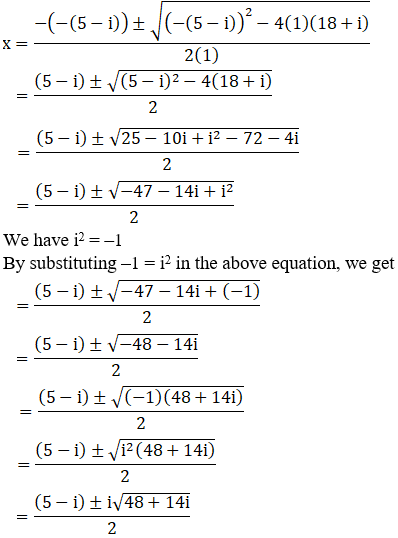We can write 48 + 14i = 49 – 1 + 14i

So,

48 + 14i = 49 + i2 + 14i [∵ i2 = –1]

= 72 + i2 + 2(7)(i)

= (7 + i)2 [Since, (a + b)2 = a2 + b2 + 2ab]

By using the result 48 + 14i = (7 + i) 2, we get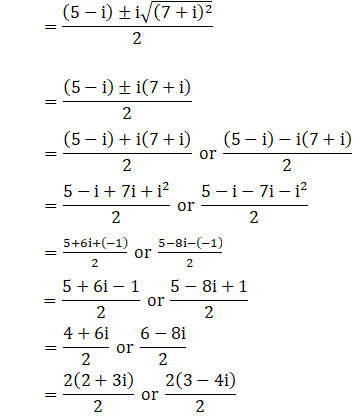x = 2 + 3i or 3 – 4i

∴ The roots of the given equation are 3 – 4i, 2 + 3i

(iii) (2 + i)x2 – (5- i)x + 2 (1 – i) = 0

Given: (2 + i)x2 – (5- i)x + 2 (1 – i) = 0

We shall apply discriminant rule,

Where, x = (-b ±√(b2 – 4ac))/2a

Here, a = (2+i), b = -(5-i), c = 2(1-i)

So,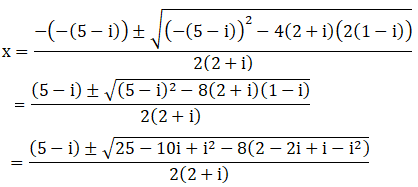We have i2 = –1

By substituting –1 = i2 in the above equation, we get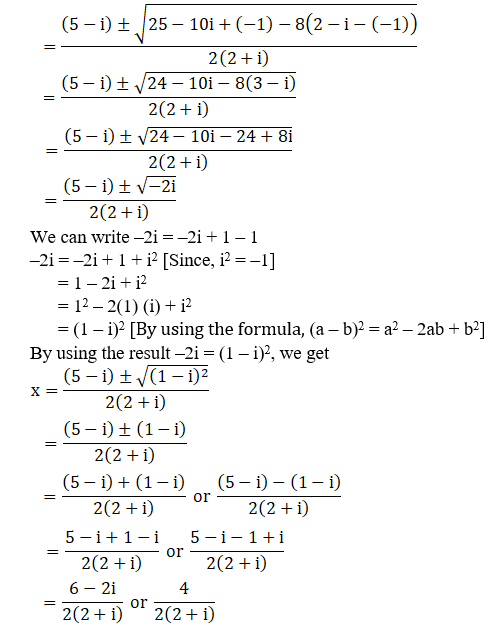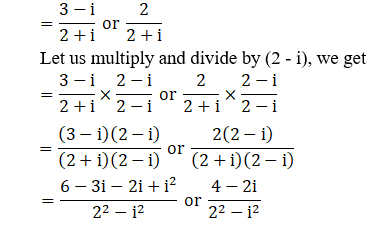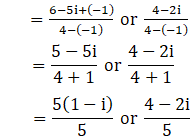x = (1 – i) or 4/5 – 2i/5

∴ The roots of the given equation are (1 – i), 4/5 – 2i/5

(iv) x2 – (2 + i)x – (1 – 7i) = 0

Given: x2 – (2 + i)x – (1 – 7i) = 0

We shall apply discriminant rule,

Where, x = (-b ±√(b2 – 4ac))/2a

Here, a = 1, b = -(2+i), c = -(1-7i)

So,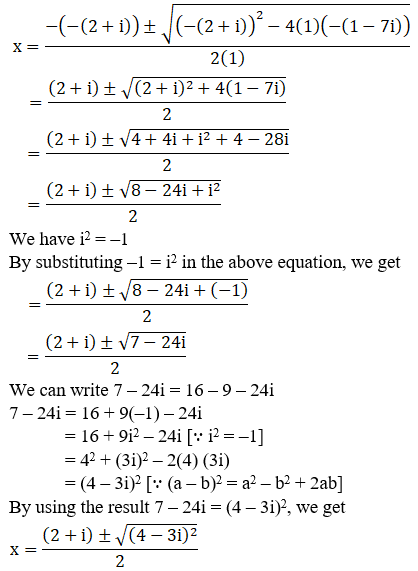We can write 7 – 24i = 16 – 9 – 24i

7 – 24i = 16 + 9(–1) – 24i

= 16 + 9i2 – 24i [∵ i2 = –1]

= 42 + (3i)2 – 2(4) (3i)

= (4 – 3i)2 [∵ (a – b)2 = a2 – b2 + 2ab]

By using the result 7 – 24i = (4 – 3i)2, we get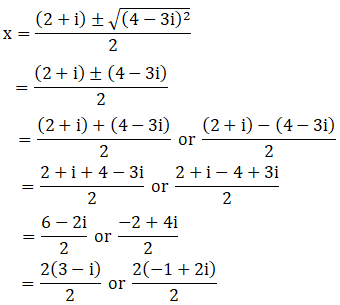x = 3 – i or -1 + 2i

∴ The roots of the given equation are (-1 + 2i), (3 – i)

(v) ix2 – 4x – 4i = 0

Given: ix2 – 4x – 4i = 0

ix2 + 4x(–1) – 4i = 0 [We know, i2 = –1]

So by substituting –1 = i2 in the above equation, we get

ix2 + 4xi2 – 4i = 0

i(x2 + 4ix – 4) = 0

x2 + 4ix – 4 = 0

x2 + 4ix + 4(–1) = 0

x2 + 4ix + 4i2 = 0 [Since, i2 = –1]

x2 + 2ix + 2ix + 4i2 = 0

x(x + 2i) + 2i(x + 2i) = 0

(x + 2i) (x + 2i) = 0

(x + 2i)2 = 0

x + 2i = 0

x = –2i, -2i

∴ The roots of the given equation are –2i, –2i

(vi) x2 + 4ix – 4 = 0

Given: x2 + 4ix – 4 = 0

x2 + 4ix + 4(–1) = 0 [We know, i2 = –1]

So by substituting –1 = i2 in the above equation, we get

x2 + 4ix + 4i2 = 0

x2 + 2ix + 2ix + 4i2 = 0

x(x + 2i) + 2i(x + 2i) = 0

(x + 2i) (x + 2i) = 0

(x + 2i)2 = 0

x + 2i = 0

x = –2i, -2i

∴ The roots of the given equation are –2i, –2i

(vii) 2x2 + √15ix – i = 0

Given: 2x2 + √15ix – i = 0

We shall apply discriminant rule,

Where, x = (-b ±√(b2 – 4ac))/2a

Here, a = 2, b = √15i, c = -i

So,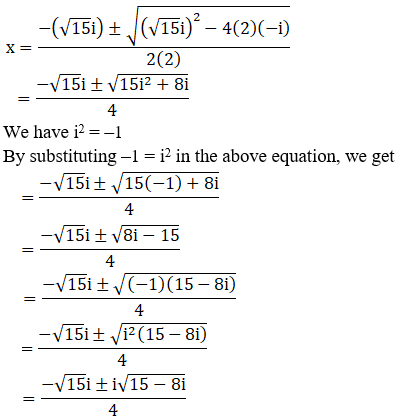We can write 15 – 8i = 16 – 1 – 8i

15 – 8i = 16 + (–1) – 8i

= 16 + i2 – 8i [∵ i2 = –1]

= 42 + (i)2 – 2(4)(i)

= (4 – i)2 [Since, (a – b)2 = a2 – b2 + 2ab]

By using the result 15 – 8i = (4 – i)2, we get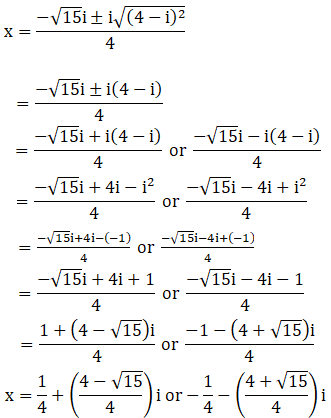∴ The roots of the given equation are [1+ (4 – √15)i/4] , [-1 -(4 + √15)i/4]

(viii) x2 – x + (1 + i) = 0

Given: x2 – x + (1 + i) = 0

We shall apply discriminant rule,

Where, x = (-b ±√(b2 – 4ac))/2a

Here, a = 1, b = -1, c = (1+i)

So,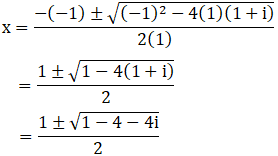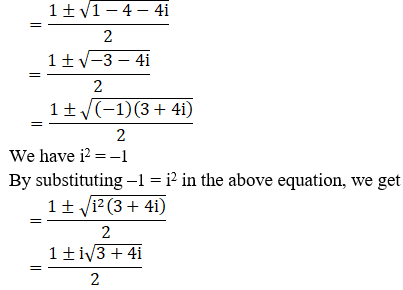We can write 3 + 4i = 4 – 1 + 4i

3 + 4i = 4 + i2 + 4i [∵ i2 = –1]

= 22 + i2 + 2(2) (i)

= (2 + i)2 [Since, (a + b)2 = a2 + b2 + 2ab]

By using the result 3 + 4i = (2 + i)2, we get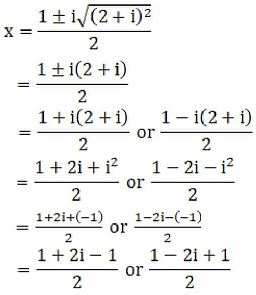x = 2i/2 or (2 – 2i)/2

x = i or 2(1-i)/2

x = i or (1 – i)

∴ The roots of the given equation are (1-i), i

(ix) ix2 – x + 12i = 0

Given: ix2 – x + 12i = 0

ix2 + x(–1) + 12i = 0 [We know, i2 = –1]

so by substituting –1 = i2 in the above equation, we get

ix2 + xi2 + 12i = 0

i(x2 + ix + 12) = 0

x2 + ix + 12 = 0

x2 + ix – 12(–1) = 0

x2 + ix – 12i2 = 0 [Since, i2 = –1]

x2 – 3ix + 4ix – 12i2 = 0

x(x – 3i) + 4i(x – 3i) = 0

(x – 3i) (x + 4i) = 0

x – 3i = 0 or x + 4i = 0

x = 3i or –4i

∴ The roots of the given equation are -4i, 3i

(x) x2 – (32 – 2i)x – 2i = 0

Given: x2 – (32 – 2i)x – 2i = 0

We shall apply discriminant rule,

Where, x = (-b ±√(b2 – 4ac))/2a

Here, a = 1, b = -(32 – 2i), c = –2i

So,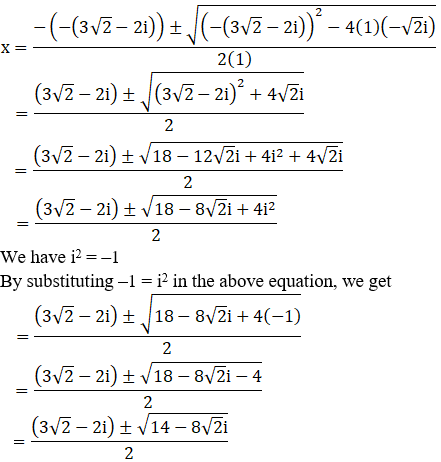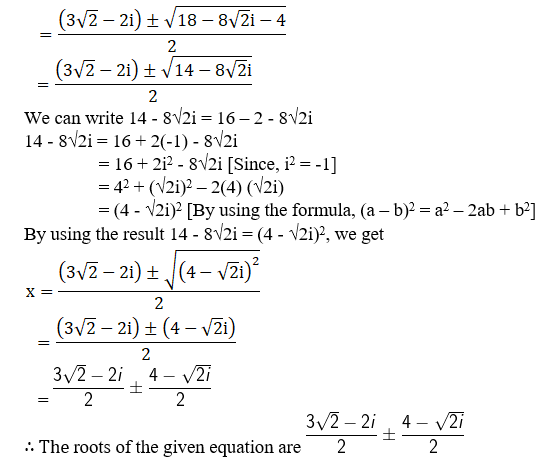(xi) x2 – (2 + i)x + 2i = 0

Given: x2 – (2 + i)x + 2i = 0

x2 – (2x + ix) + 2i = 0

x22x – ix + 2i = 0

x(x – 2) – i(x – 2) = 0

(x – 2) (x – i) = 0

(x – 2) = 0 or (x – i) = 0

x = 2 or x = i

∴ The roots of the given equation are i, 2

(xii) 2x2 – (3 + 7i)x + (9i – 3) = 0

Given: 2x2 – (3 + 7i)x + (9i – 3) = 0

We shall apply discriminant rule,

Where, x = (-b ±√(b2 – 4ac))/2a

Here, a = 2, b = -(3 + 7i), c = (9i – 3)

So,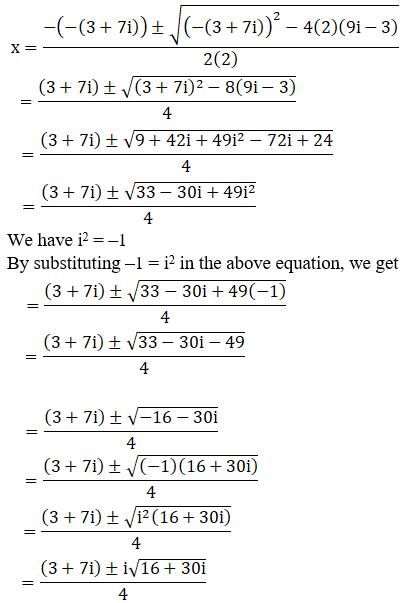We can write 16 + 30i = 25 – 9 + 30i

16 + 30i = 25 + 9(–1) + 30i

= 25 + 9i2 + 30i [∵ i2 = –1]

= 52 + (3i)2 + 2(5)(3i)

= (5 + 3i)2 [∵ (a + b)2 = a2 + b2 + 2ab]

By using the result 16 + 30i = (5 + 3i)2, we get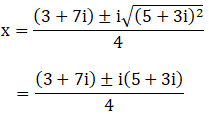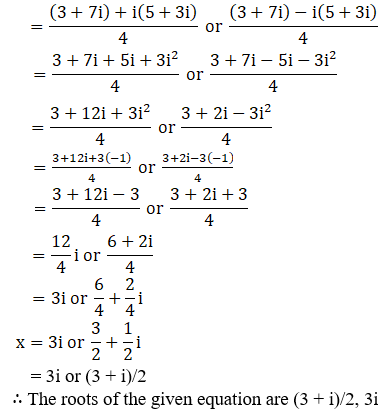### Also, access exercises of RD Sharma Solutions for Class 11 Maths Chapter 14 – Quadratic Equations

Exercise 14.1 Solutions

Exercise 14.2 Solutions

## Frequently Asked Questions on RD Sharma Solutions for Class 11 Maths Chapter 14

### What are the ways to learn the topics from RD Sharma Solutions for Class 11 Maths Chapter 14 faster?

Students can quickly comprehend the key topics by referring to the RD Sharma Solutions for Class 11 Maths Chapter 14. It comprises solutions to textbook questions written in an elaborate manner highlighting the important points. Further, all the solutions are formulated by subject experts as per the latest CBSE syllabus and guidelines.

### How should I prepare for the board exam using the RD Sharma Solutions for Class 11 Maths Chapter 14?

Students can refer to CBSE syllabus and the correct study materials like the RD Sharma Solutions provided at BYJU’S should be chosen to score good marks in the exam. Students need to learn the chapter and revise them on a regular basis to get a grip on the concepts. Students should refer to the solutions while learning a new chapter to understand the method of answering questions as per the latest CBSE guidelines. The syllabus for the academic year should be understood before the exam to know the marks weightage of each concept covered in the chapter.

### Can the RD Sharma Solutions for Class 11 Maths Chapter 14 really help students with their board exam preparations?

The Class 11 board exams are crucial in every student’s academic life as it lays a foundation for all career goals. The resource primarily increases the logical and analytical thinking skills of students which are vital for taking an exam. Before kickstarting preparations for the exam, students must have an overview of the syllabus and mark weightage for the concepts as per the CBSE guidelines. The solutions are prepared by subject matter experts at BYJU’S with an aim to clarify doubts of students while learning the solutions to the textbook questions.